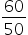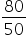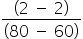Mathematics
Easy

Question

# There are 240 seniors in Kathryn’s school. Her class is planning a trip and is taking buses that hold a maximum of 50 passengers. Assume that the trip is optional.what is the average rate of change of the function over the interval from 60 to 80?

## 00.051.251Hint:

## The correct answer is: 0

### x = 60, f(x) = ceiling() = ceiling(1.2) = 2 x = 80, f(x) = ceiling() = ceiling(1.6) = 2 Rate of change (m) == 0.Hence, the correct option is D.

The ceiling function is also known as the smallest integer function and the floor function is also known as the greatest integer function.

### Related Questions to study#### With Turito Foundation.#### Get an Expert Advice From Turito.